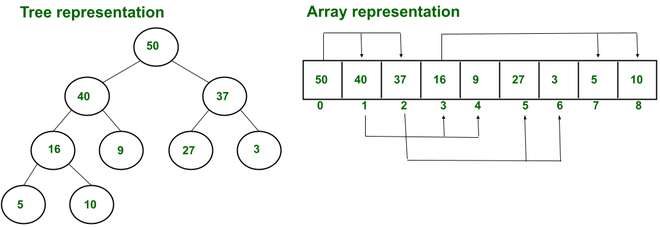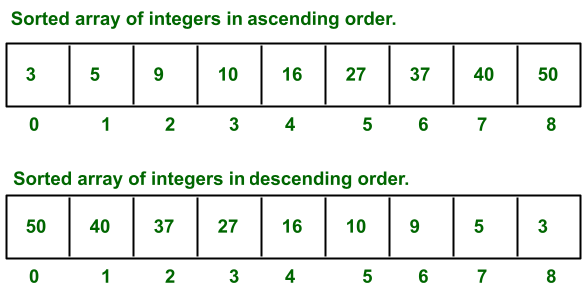Open In App

# Difference between Heaps and Sorted Array

1. Heap:
A heap is a tree based data structure in which tree should be almost complete. It is of two types i.e. max and min heap.

• Max heap: In max heap, if p is the parent and c is its child, then for every parent p the value of it is greater than or equal to the value of c
• Min heap In min heap, if p is the parent and c is its child, then for every parent p value is less than or equal to the value of c.

Heap is also used as priority queue. In which highest(in case of max heap) or lowest(in case of min heap) element is present at the root.
Heap is used in problems where we need to remove the highest or lowest priority element. A common implementation of heap is binary heap.

Implementation

• Although heap can be implemented as a tree, but lots of storage will go waste for storing pointers. Due to the property of heap being a complete binary tree, it can be easily stored in an array.
• Where root element is stored at first index and its child index can be calculated as following.
• Left child index = 2×r  where r is index of the root and array starting index is 1 .
• Right child index = 2×r+1.
• And parent index can be calculated as floor (i/2) where i is the index of its left or right child.

Example:Example of Max heap

2. Sorted array:
A sorted array is a data structure in which elements are sorted in numerical, alphabetical, or by some other order and stored at contiguous memory locations.Sorted array example

• All data structures have their own pros and cons depending on the problems or algorithms where they are used. For example heap is the best data structure in situations where we need optimality in finding (max or min frequently), deleting (max or min) or inserting (max or min) element.
• And sorted array is used in situations when items need to be stored in ascending or descending orders. For example: in shortest-job-first scheduling algorithms where the processes(stored in array) need to be sorted according to burst time of the processes. So in case sorting array is required.

Difference between Heaps and Sorted Array: